# Specifying FFTs directly

#### 2017-11-01

There are two ways to define fast-and-frugal trees manually when using the FFTrees() function, either as a sentence using the my.tree argument (the easier way), or as a dataframe using the tree.definitions argument (the harder way). Both of these methods will bypass the tree construction algorithms built into FFTrees.

### my.tree

The first method is to use the my.tree argument, where my.tree is a sentence describing a (single) FFT. When this argument is specified in FFTrees(), the function (specifically wordstoFFT() will try to extract the specified FFT from the argument.

For example, let’s look at the columns sex, age and thal in the heartdisease data:

head(heartdisease[c("sex", "age", "thal")])
##   sex age   thal
## 1   1  63     fd
## 2   1  67 normal
## 3   1  67     rd
## 4   1  37 normal
## 5   0  41 normal
## 6   1  56 normal

Here’s how we could specify an FFT using these cues as a sentence:

my.tree = "If sex = 1, predict True.
If age < 45, predict False.
If thal = {fd, normal}, predict True. Otherwise, predict False"

Here are some notes on specifying trees manually:

• Each node must start with the word “If” and should in the form: If CUE DIRECTION THRESHOLD, predict EXIT.
• Numeric thresholds shold be specified directly (without brackets).
• Factor thresholds must be specified within braces like sex = {male}. For factors with sets of values, values within a threshold should be separated by commas like eyecolor = {blue,brown}
• Standard logical comparisons =, !=, <, >= (etc.) are valid. For numeric cues, only use >, >=, <, <=. For factors, only use = and !=.
• Positive exits are indicated by True, while negative exits are specified by False. The final node will be forced to have a bidirectional exit. The text Otherwise, predict EXIT I’ve included in the example above is actually not necessary.

Now, let’s pass the my.tree argument to FFTrees() to force apply our FFT to the heartdisease data:

# Pass a verbally defined FFT to FFTrees with the my.tree argument
my.heart.fft <- FFTrees(diagnosis ~.,
data = heartdisease,
my.tree = "If sex = 1, predict True.
If age < 45, predict False.
If thal = {fd, normal}, predict True.
Otherwise, predict False")

Let’s see how well our FFT did:

# Plot
plot(my.heart.fft)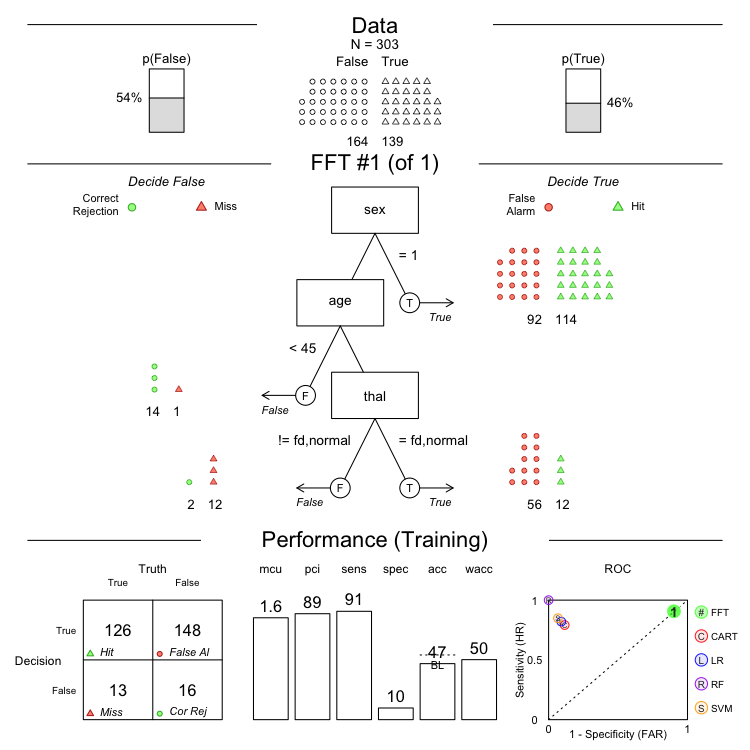As you can see, this FFT is pretty terrible – it has a high sensitivity, but a terrible specificity.

Let’s see if we can come up with a better one using the cues thal, cp, and ca

# Specify an FFt verbally with the my.tree argument
my.heart.fft <- FFTrees(diagnosis ~.,
data = heartdisease,
my.tree = "If thal = {rd,fd}, predict True.
If cp != {a}, predict False.
If ca > 1, predict True.
Otherwise, predict False")

# Plot
plot(my.heart.fft)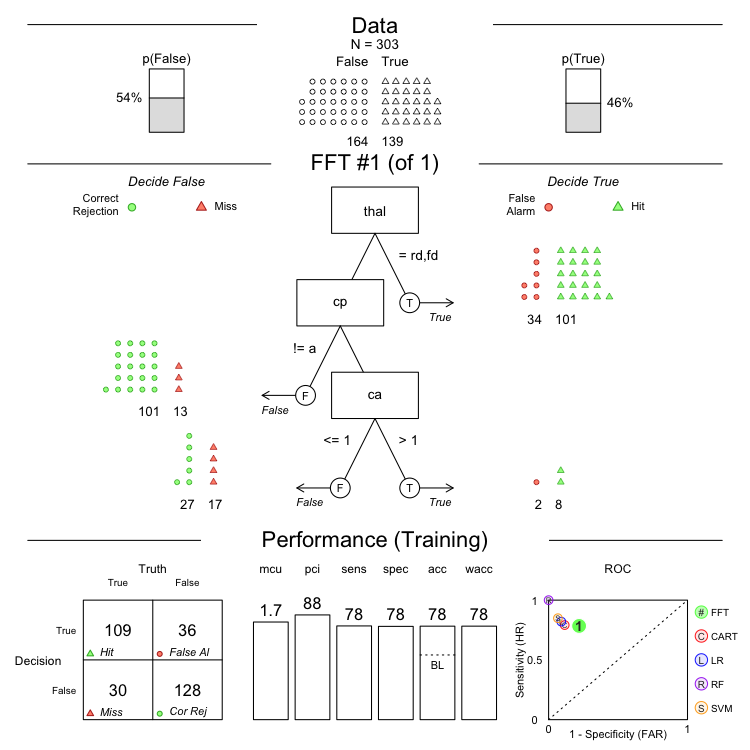This one looks much better!

Here’s one more example using the titanic data. We’ll create an FFT predicting whether a person survived the Titanic using the cues class, age, and sex:

head(titanic[c("class", "age", "sex", "survived")])
##   class   age  sex survived
## 1 first adult male        1
## 2 first adult male        1
## 3 first adult male        1
## 4 first adult male        1
## 5 first adult male        1
## 6 first adult male        1
my.titanic.tree <- "If age = {child}, predict True.
If sex = {female}, predict True.
If class = {first}, predict True.
Otherwise, predict False"

titanic.fft <- FFTrees(survived ~.,
data = titanic,
my.tree = my.titanic.tree,
comp = FALSE)
## Warning in FFTrees(survived ~ ., data = titanic, my.tree =
## my.titanic.tree, : The argument comp is depricated. Use do.comp instead.
plot(titanic.fft)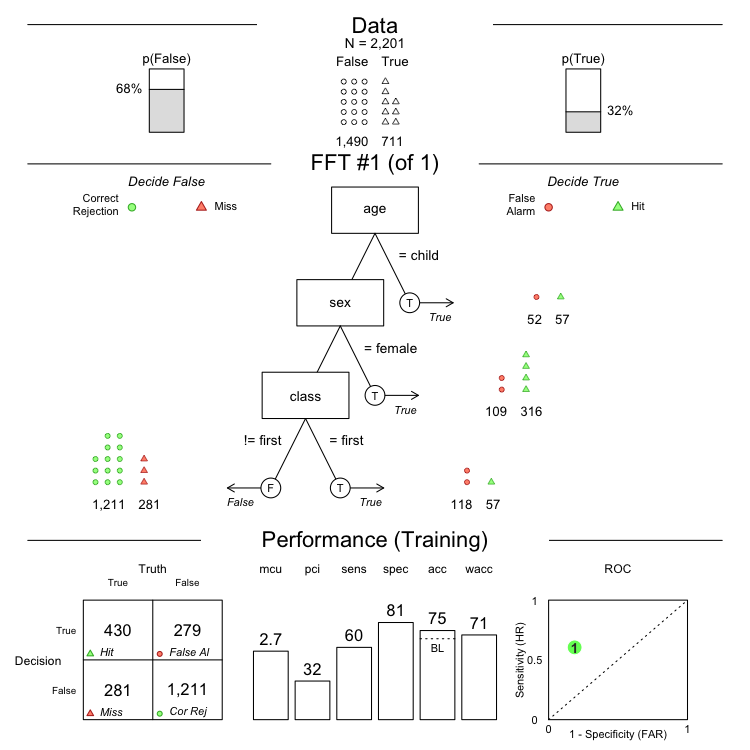### tree.definitions

The second way to define one (or more) fast-and-frugal trees is with the tree.definitions argument. This argument should be a dataframe with the following structure:

##   tree nodes classes               cues directions    thresholds     exits
## 1    1     3   c;c;n         thal;cp;ca      =;=;>     rd,fd;a;0   1;0;0.5
## 2    2     4 c;c;n;n thal;cp;ca;thalach    =;=;>;< rd,fd;a;0;148 1;0;1;0.5
## 3    3     3   c;c;n         thal;cp;ca      =;=;>     rd,fd;a;0   0;1;0.5
## 4    4     4 c;c;n;n thal;cp;ca;thalach    =;=;>;< rd,fd;a;0;148 1;1;0;0.5
## 5    5     4 c;c;n;n thal;cp;ca;thalach    =;=;>;< rd,fd;a;0;148 0;0;1;0.5
## 6    6     4 c;c;n;n thal;cp;ca;thalach    =;=;>;< rd,fd;a;0;148 1;1;1;0.5
## 7    7     4 c;c;n;n thal;cp;ca;thalach    =;=;>;< rd,fd;a;0;148 0;0;0;0.5

The dataframe should have 7 columns:

1. tree: An indexing integer
2. nodes: The number of nodes in the tree.

The following 5 columns define each node in an FFT, where nodes are separated by semi-colons ;:

1. classes: The class of each node in the tree. c = character, n = numeric, i = integert.
2. cues: The names of the cues
3. directions: The direction of positive decisions for that cue. Even if a cue only has a negative exit branch, the direction should always be specified as if it was making a positive decision.
4. thresholds: The decision threshold for the cue. For numeric cues, thresholds are single numbers. For factor cues, they are sets of factor values (separted by commas)
5. exits: The exit direction for the cue. 0 = negative exit, 1 = positive exit, .5 = both a negative and a positive exit (only for the final node in a tree)

On can see examples of tree.definitions dataframes in an FFTrees object. For example, the definitions above can be obtained as follows:

# Create an FFTrees object
heart.fft <- FFTrees(diagnosis ~.,
data = heartdisease)

# Get the tree definitions
heart.tree.definitions <- heart.fft\$tree.definitions

# Print the result
heart.tree.definitions
##   tree nodes classes               cues directions    thresholds     exits
## 1    1     3   c;c;n         thal;cp;ca      =;=;>     rd,fd;a;0   1;0;0.5
## 2    2     4 c;c;n;n thal;cp;ca;thalach    =;=;>;< rd,fd;a;0;148 1;0;1;0.5
## 3    3     3   c;c;n         thal;cp;ca      =;=;>     rd,fd;a;0   0;1;0.5
## 4    4     4 c;c;n;n thal;cp;ca;thalach    =;=;>;< rd,fd;a;0;148 1;1;0;0.5
## 5    5     4 c;c;n;n thal;cp;ca;thalach    =;=;>;< rd,fd;a;0;148 0;0;1;0.5
## 6    6     4 c;c;n;n thal;cp;ca;thalach    =;=;>;< rd,fd;a;0;148 1;1;1;0.5
## 7    7     4 c;c;n;n thal;cp;ca;thalach    =;=;>;< rd,fd;a;0;148 0;0;0;0.5

One can use tree.definitions dataframes created from FFTrees() as a template, make adjustments, and then feed the dataframe back into FFTrees() to create new, customized trees. Below, I’ll create definitions of two FFTs, then pass them to FFTrees(). The two FFTS can be described as follows.

• Tree #1 “If slope != {down, up}, then predict False. If ca is greater than 1, predict True. Otherwise, predict False”
• Tree #2 “If chol < 300, then predict True If oldpeak is greater than 2, predict True. If restecg is not normal, then predict False. Otherwise, predict True”
# Define two trees
my.tree.definitions <- data.frame(tree = c(1, 2),
nodes = c(2, 3),
classes = c("c;n", "n;n;f"),
cues = c("slope;ca", "chol;oldpeak;restecg"),
directions = c("=;>", "<;>;!="),
thresholds = c("down,up;1", "300;2;normal"),
exits = c("0;.5", "1;1;.5"),
stringsAsFactors = FALSE)

Now, we can pass these trees to FFTrees() and view their resulting performance:

#Pass trees to FFTrees with tree.definitions
my.heart.fft <- FFTrees(diagnosis ~ .,
data = heartdisease,
tree.definitions = my.tree.definitions)

# Show summary statistics
my.heart.fft
## FFT #1 predicts diagnosis using 2 cues: {slope,ca}
##
##  If slope != {down,up}, predict False.
##  If ca <= 1, predict False, otherwise, predict True.
##
##                    train
## cases       :n    303.00
## speed       :mcu    1.54
## frugality   :pci    0.89
## accuracy    :acc    0.57
## weighted    :wacc   0.53
## sensitivity :sens   0.12
## specificity :spec   0.95
##
## pars: algorithm = 'ifan', goal = 'wacc', goal.chase = 'bacc', sens.w = 0.5, max.levels = 4
# Plot Tree 2
plot(my.heart.fft, tree = 2)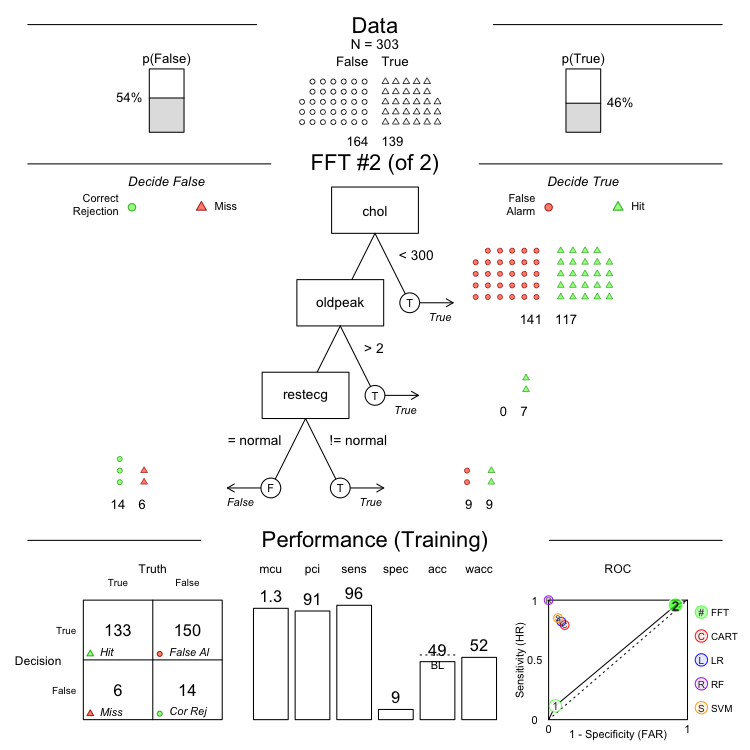Here is Tree #1: “If slope != {down, up}, then predict False. If ca is greater than 1, predict True. Otherwise, predict False”

# Plot Tree 1
plot(my.heart.fft, tree = 1)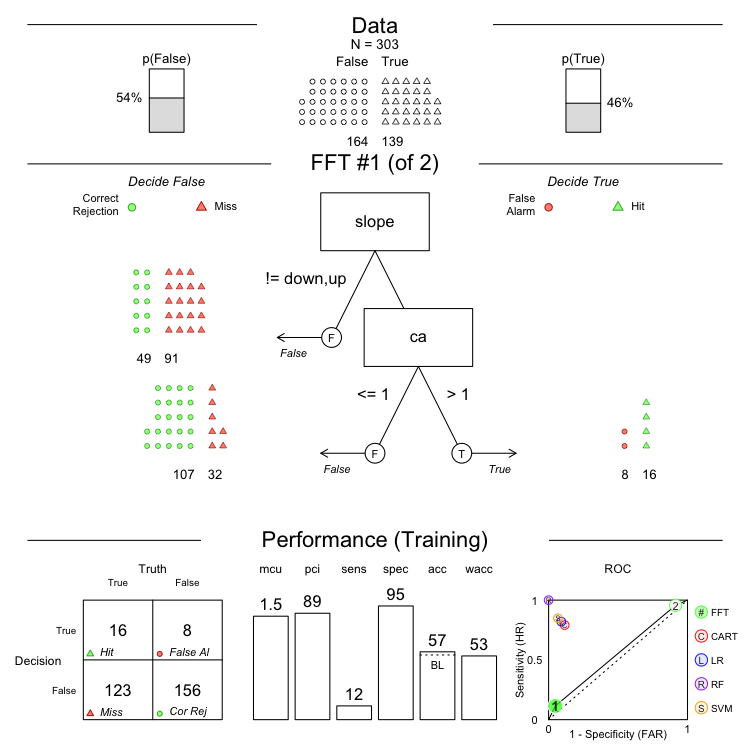Here is Tree #2: “If chol < 300, then predict True. If oldpeak is greater than 2, predict True. If restecg is not normal, then predict False. Otherwise, predict True”

# Plot Tree 2
plot(my.heart.fft, tree = 2)